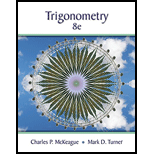Chapter 6, Problem 8CTTrigonometry (MindTap Course List)

8th Edition
Charles P. McKeague + 1 other
ISBN: 9781305652224

Solutions

Chapter
SectionTrigonometry (MindTap Course List)

8th Edition
Charles P. McKeague + 1 other
ISBN: 9781305652224
Textbook Problem

Find all solutions in the interval 0 ° ≤ θ ≤ 360 ° . If rounding is necessary, round to the nearest tenth of a degree. 4 cos 2 θ + 2 sin θ = 1

3
To determine

To calculate:

All the solutions of the given trigonometric equation.

Explanation

Given information:

4cos2θ+2sinθ=1

Concept Used:

To find the solution of the equation we factorise the equation by taking out common terms.

Also use identity cos2θ=12sin2θ to write each term in same trigonometric function

Calculation:

The given equation is:

4cos2θ+2sinθ=1

Using cos2θ=12sin2θ

4(12sin2θ)+2sinθ=148sin2θ+2sinθ1=0

On simplifying we get

8sin2θ2sinθ3=0

Now factorise the equation

8sin2θ(64)sinθ3=08sin2θ6sinθ+4sinθ3=02sinθ

Still sussing out bartleby?

Check out a sample textbook solution.

See a sample solution

The Solution to Your Study Problems

Bartleby provides explanations to thousands of textbook problems written by our experts, many with advanced degrees!

Get Started

Describe the scores in a sample that has standard deviation of zero.

Statistics for The Behavioral Sciences (MindTap Course List)

Calculate y'. 38. y=arctan(arcsinx)

Single Variable Calculus: Early Transcendentals

The length of the are r = e for 0 is given by: a) 0ed b) 02e2d c) 02ed d) 02ed

Study Guide for Stewart's Single Variable Calculus: Early Transcendentals, 8th